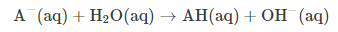# IMAT 2011 Q65 [Reactions]

Which one of the following is not an acid/base reaction?

A. {HNO_3 + HClO_4 → H2NO3^+ + ClO4^-}
B. {2NH_3 → NH_2^– + NH_4^+}
C. {Al(H_2O)_6 ^{3+} + H_2O → Al(H_2O)_5(OH)^{2+} + H_3O^+}
D. {CH_4 + H(SbF_6) → CH_5^+ + SbF_6^–}
E. {FeCl_3 + 6H_2O → Fe(H_2O)_6 ^{3+} + 3Cl^-}

To answer this question we have to know what the definition of an acid and base is according to Bronsted-Lowry.

An acid is any substance that is a proton donor and the proton acceptor is the conjugate base. Thus, searching for an acid and its conjugate base in each of the choices can give us the answer, E.

Here are some characteristics of acid and bases that are very important to remember for the IMAT exam, as mentioned in the free IMAT course on the website.

### Acids

Acids have long been recognized as a distinctive class of compounds whose aqueous solutions exhibit the following properties:

• A characteristic sour taste.
• Changes the color of litmus from blue to red.
• Reacts with certain metals to produce gaseous H2.
• Reacts with bases to form a salt and water.

Acidic solutions have a pH less than 7, with lower pH values corresponding to increasing acidity. Common examples of acids include acetic acid (in vinegar), sulfuric acid (used in car batteries), and tartaric acid (used in baking).

There are three common definitions for acids:

• Arrhenius acid: any substances that increases the concentration of hydronium ions (H3O+) in solution.
• Brønsted-Lowry acid: any substance that can act as a proton donor.
• Lewis acid: any substance that can accept a pair of electrons.

### Acid Strength and Strong Acids

The strength of an acid refers to how readily an acid will lose or donate a proton, oftentimes in solution. A stronger acid more readily ionizes, or dissociates, in a solution than a weaker acid. The six common strong acids are:

• hydrochloric acid (HCl)
• hydrobromic acid (HBr)
• hydroiodic acid (HI)
• sulfuric acid (H2SO4; only the first proton is considered strongly acidic)
• nitric acid (HNO3)
• perchloric acid (HClO4)

Each of these acids ionize essentially 100% in solution. By definition, a strong acid is one that completely dissociates in water; in other words, one mole of the generic strong acid, HA, will yield one mole of H+, one mole of the conjugate base, A−, with none of the unprotonated acid HA remaining in solution. By contrast, however, a weak acid, being less willing to donate its proton, will only partially dissociate in solution. At equilibrium, both the acid and the conjugate base will be present, along with a significant amount of the undissociated species, HA.

### Factors Affecting Acid Strength

Two key factors contribute to overall strength of an acid:

• polarity of the molecule
• strength of the H-A bond

These two factors are actually related. The more polar the molecule, the more the electron density within the molecule will be drawn away from the proton. The greater the partial positive charge on the proton, the weaker the H-A bond will be, and the more readily the proton will dissociate in solution.

Acid strengths are also often discussed in terms of the stability of the conjugate base. Stronger acids have a larger Ka and a more negative pKa than weaker acids.

### Bases

There are three common definitions of bases:

• Arrhenius base: any compound that donates an hydroxide ion (OH–) in solution.
• Brønsted-Lowry base: any compound capable of accepting a proton.
• Lewis base: any compound capable of donating an electron pair.

In water, basic solutions will have a pH between 7-14.

### Base Strength and Strong Bases

A strong base is the converse of a strong acid; whereas an acid is considered strong if it can readily donate protons, a base is considered strong if it can readily deprotonate (i.e, remove an H+ ion) from other compounds. As with acids, we often talk of basic aqueous solutions in water, and the species being deprotonated is often water itself. The general reaction looks like:Thus, deprotonated water yields hydroxide ions, which is no surprise. The concentration of hydroxide ions increases as pH increases.

Most alkali metal and some alkaline earth metal hydroxides are strong bases in solution. These include:

• sodium hydroxide (NaOH)
• potassium hydroxide (KOH)
• lithium hydroxide (LiOH)
• rubidium hydroxide (RbOH)
• cesium hydroxide (CsOH)
• calcium hydroxide (Ca(OH)2)
• barium hydroxide (Ba(OH)2)
• strontium hydroxide (Sr(OH)2)

The alkali metal hydroxides dissociate completely in solution. The alkaline earth metal hydroxides are less soluble but are still considered to be strong bases.